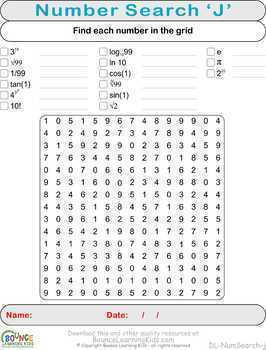# Numbers search (12 distance learning worksheets for Numeracy)Subject
Resource Type
File Type
Zip (7 MB|12 pages)
\$4.95
Also included in:
1. A fantastic selection of fun math games and puzzles all in a single bundle.Each of the numeracy products included in this bundle provides a fun and distracting way for kids to exercise all manner of numeracy related activities, such as:Basic arithmetic (addition, subtraction, division, multiplicatio
\$54.45
\$35.95
Save \$18.50
2. A fantastic selection of fun math games and puzzles all in a single bundle.Each of the numeracy products included in this bundle provides a fun and distracting way for kids to exercise all manner of numeracy related activities, such as:Basic arithmetic (addition, subtraction, division, multiplicatio
\$60.45
\$39.95
Save \$20.50
3. A fantastic selection of fun math games and puzzles all in a single bundle.Each of the numeracy products included in this bundle provides a fun and distracting way for kids to exercise all manner of numeracy related activities, such as:Basic arithmetic (addition, subtraction, division, multiplicatio
\$60.45
\$39.95
Save \$20.50
4. A fantastic selection of fun math games and puzzles all in a single bundle.Each of the math products included in this bundle provides a fun and distracting way for kids to exercise all manner of numeracy related activities, such as:Basic arithmetic (addition, subtraction, division, multiplication)Fa
\$60.45
\$39.95
Save \$20.50
Product Description

Numbers search is a fantastic way to make mental maths fun. Just like a regular wordsearch puzzle but with numbers instead of letters.

As an added twist, some of the numbers you need to look for must first be determined by solving a basic equation. There are different sizes of grids and equations for different ability levels.

• Grid A - 8x8 numbers
Search for the numbers as depicted. The first two are already highlighted to help show how it works.
• Grid B - 8x8 numbers
Search for the numbers as depicted. No hints this time.
• Grid C - 8x8 grid
Solve the ADDITION equations to determine which numbers to find and then find them in the grid. One solution has been highlighted to help show how it works.
• Grid D - 8x8 grid
Solve the SUBTRACTION equations to determine which numbers to find and then find them in the grid. No hints this time.
• Grid E - 8x8 grid
Solve the MULTIPLICATION equations to determine which numbers to find and then find them in the grid. One solution has been highlighted to help show how it works.
• Grid F - 8x8
Solve the DIVISION equations to determine which numbers to find and then find them in the grid. One solution has been highlighted to help show how it works.
• Grid G - 10x10 grid (larger grids are harder to solve)
Solve the ADDITION equations to determine which numbers to find and then find them in the grid. This time, there are three numbers to sum instead of two on the easier grid. One solution has been highlighted to help show how it works.
• Grid H - 10x10 grid
Solve the SUBTRACTION equations to determine which numbers to find and then find them in the grid. No hints this time.
• Grid I - 10x10 grid
Solve the MULTIPLICATION equations to determine which numbers to find and then find them in the grid. No hints this time.
• Grid J - 15-15 grid
This time, instead of solving specific equations, have fun exploring the features on your scientific calculator by finding various mathematical constants and other fun functions, like Pi, Log, Root, Factorial, Sin, Cos, Tan, etc. Figure out which numbers each function represents and then find it in the grid - especially fun for those keen with numbers.

Suitable for printing in both color and B/W.

Ideal for distance learning - no prep required!

Each worksheet is presented as an individual JPG but there is also a single PDF that contains all the worksheets together, so you have the best of both worlds to choose from.

Free TPT credits:

Go to the My Purchases page and find the Provide Feedback button. Use that to leave a rating/comment. Each time you do this, TPT will give you credits towards future purchases. As an added bonus, the feedback will help me to improve my products :-)

Half price:

Scroll to the top of this page and click on the green star just below the Bounce Learning Kids store name. You'll be able to get new products for half off in the first 24 hours of release!

FREE products:

More quality Visual Learning Resources from Bounce Learning Kids:

Total Pages
12 pages